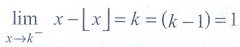# Chapter 9: Differential Calculus Limits and Continuity - Online Test

Q1. Let the function f be defined by f (x)=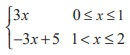, then
Explaination / Solution:Q2. If f : R→ R  is defined by f ( x)=+ |x- 4| for x R, then limx→3- f (x) is equal to
Explaination / Solution:
No Explaination.

Q3.is
Explaination / Solution: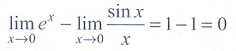Q4. If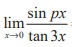= 4 , then the value of p is
Explaination / Solution:
No Explaination.

Q5.is
Explaination / Solution:
No Explaination.

Q6.is
Explaination / Solution: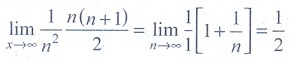Q7.Explaination / Solution:
No Explaination.

Q8.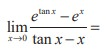Explaination / Solution:
No Explaination.

Q9. The value of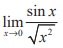is
Explaination / Solution:
No Explaination.

Q10. The value of, where k is an integer is
Explaination / Solution: